# DSP Voice Frequency Compander

for use in RF Communications

Published in QEX, July 1994

John Ash, KB7ONG, jash@eecs.wsu.edu
Fred Christensen, KA6PNW, fchriste@eecs.wsu.edu
Rob Frohne, KL7NA, My email address is my first name "dot" my last name "at sign" wallawalla.edu.

## Introduction

With the radio frequency (RF) spectrum getting more and more crowded all the time there is a profound need to make more efficient use of these bands. In 1978, Harris and Cleveland  developed a unique method of voice frequency and amplitude companding called narrow band voice modulation (NBVM). This technique offered significant improvement in bandwidth conservation, which in turn improved signal to noise ratio (SNR) and decreased co-channel interference. However, their technique required cumbersome analog hardware. With new DSP hardware it is now possible to develop these techniques in a flexible and fairly inexpensive way. This project involves implementing the voice frequency companding part of Harris and Cleveland's NBVM on Texas Instrument's new DSP Starter Kit (DSK).

Simply put, frequency companding is a method of removing the most unused frequency components from a given source and then shifting the remaining components together. The resulting signal may be sent over nearly any analog or digital transmission system. On the receiving end, the frequency components are expanded back out before the signal is used. With the technique described in this article, the bandwidth necessary for intelligible speech can be reduced from about 3 kHz down to about 1.7 kHz, thus conserving over 40% in RF bandwidth usage. In addition, the SNR is improved by about 2.4 dB and co-channel interference is reduced.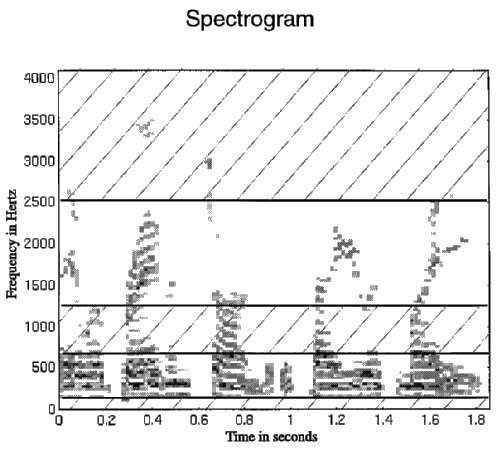Fig 1 - Spectrogram of a male voice saying: "They were brave and strong, but the tiger was mighty". In a spectrogram, the presence of frequency components over a small time interval are plotted in Hertz as a function of time.

In normal speech Harris and Cleveland found that intelligible speech can be maintained with certain bands of frequencies removed. A spectrogram of a voice sample (Figure 1) shows frequency components at different times throughout a sampling period. The frequency components are calculated over small time intervals using a Fast Fourier Transform (FFT). Darker areas indicate higher energy. It is obvious that frequencies below 100 Hz and above 2500 Hz are used very little in human speech. Additionally, notice the frequencies from about 600 Hz to 1200 Hz. Harris and Cleveland found that after removing these frequency components, normal speech was still intelligible! Part of their companding technique was to simply remove this band of frequency components as well as those below 100 Hz and above 2500 Hz. All the remaining frequency components were then shifted together, ready for transmission.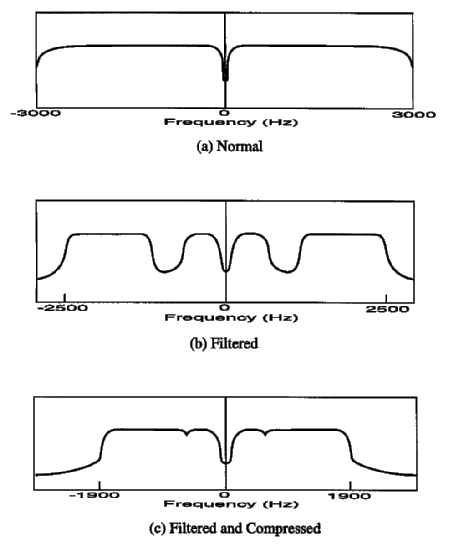Fig 2 - Audio frequency response plots for various stages in the companding process. (a) Typical voice audio signal. (b) Audio signal after removing unwanted frequencies. (c) Audio signal after remaining frequency components have been shifted together.

This can be seen in another way by looking at the audio frequency response plots shown in Figure 2. A normal audio signal usually contains all the frequencies up to 3000 Hz, except for some of the very low frequencies (Figure 2a). In this companding technique we filter out all the frequencies above 2500 Hz and those between 625 Hz and 1250 Hz. The filtered frequency response is shown in Figure 2b. Notice that the resulting gaps are basically wasted space because they would be inconvenient to use. Therefore, we shift the upper frequencies (1250 Hz to 2500 Hz) down next to the lower frequencies so we have a compressed signal looking like Figure 2c. This filtered and compressed signal can then be transmitted over any audio channel. It would be difficult to understand this signal by simply listening to it since the frequency components are not in their proper places. Therefore, on the receiving end we will just shift the upper band of frequencies back up to their original places, so that the resulting signal will again look like Figure 2b. This final signal is still quite understandable.

## System Overview

A complete companding system consists of two parts, a transmit encoder (Figure 3) and a receive decoder (Figure 4). First, the encoder takes an audio signal from a microphone, digitizes it with an A/D converter, and presents it to two specially designed digital filters (Filter #1 and #2) which eliminate all but the most important frequency components. Through Harris and Cleveland's research and some experimentation of our own, we decided the most important frequency components to keep were those from 176 Hz to 626 Hz and from 1251 Hz to 2500 Hz. In the next step, the upper pass-band (output from Filter #2T) is frequency-shifted down and added to the lower one (output from Filter #1) to make a single baseband signal with no spectral gaps which is then transmitted.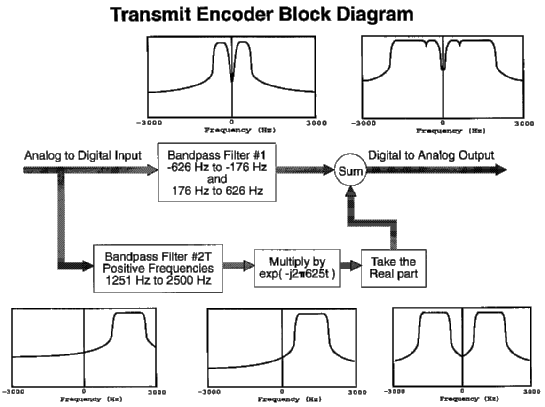Fig 3 - Block diagram of the transmit encoder section of the compander.

The decoder on the receiving end uses similar filters for re-separating the two bands. In fact, the same filter (Filter #1) can be used for the lower band, but a different bandpass filter (Filter #2R) from 626 Hz to 1875 Hz must be used for the upper band because the frequency components were previously shifted down by the encoder. The final step on the receiver end is to shift the upper pass-band (output from Filter #2R) back up to where it originally was, add it to the lower band (output from Filter #1), and send it to an audio amplifier and speaker. Though the concepts sound simple, and they are, we need to develop some theory in order to implement them.Fig 4 - Block diagram of the receive decoder section of the compander.

## Theory

There are only two basic functions that are needed: filtering and frequency shifting. For the filtering, we need two different types of bandpass filters. The first one, used both for transmitting and receiving, extracts the lower frequency components which do not need to be shifted (Figure 5, block 1). The second filter type (Figure 5, block 2T and 2R) extracts only the positive frequencies from the higher band, allowing them to be shifted. This is explained in more detail below. For our digital filters, we chose to use finite impulse response (FIR) filters (see FIR filter sidebar). These can be easily implemented with a buffer of prior inputs and a series of multiply and add operations ,  for which the DSP is optimized. Additionally, we applied Kaiser windows  with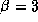to each of our FIR filters to reduce the stop-band ripple.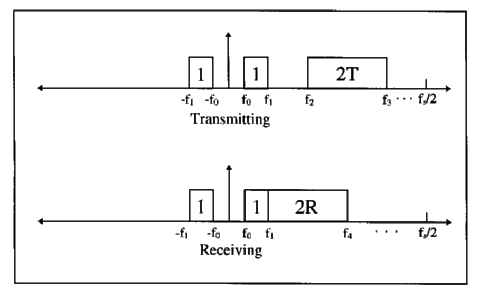Fig 5 - This shows the desired FIR filter bands. Block 1 is output of Filter #1. Block 2T is the output of Filter #2T (T=Transmit). Block 2R is the output of Filter #2R (R=Receive). Note that Filter #2T and #2R only pass positive frequencies for shifting purposes.

FIR Filter #1

For the lower pass-band between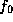and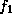, a standard bandpass FIR filter can be used. Since the frequency response of an FIR filter is periodic with period of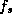(see FIR filter sidebar), the unwindowed coefficients may then be calculated by the following formula: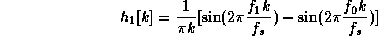(Eq. 1)

whereis the sampling frequency, and k is the tap number.

FIR Filter #2 and the Analytic Signal

Before we derive the tap coefficients for the second filter, we need to understand a mathematical concept called the analytic signal . This tool is needed for proper frequency shifting (described in the next section).

An analytic signal is a mathematical abstraction--a complex signal which contains only positive frequency components. These components can be shifted to the desired location and the result is still an analytic signal provided they weren't shifted into the negative frequency range. Since all the required information is contained in the analytic signal, the negative frequencies can be easily restored. An analytic signal, c[t], is defined to be of the form

c[t] = a[t] - jb[t] (Eq. 2)

where t is time, a[t] is the original signal including both the positive and negative frequency components, and b[t] is its Hilbert transform. To restore the original signal from an analytic signal, we simply take the real part (i.e. drop the imaginary part, b[t]).

So, how is this analytic signal created? Well, it is created by simply filtering out the negative frequency components. Since we also need to filter the pass-band (the one that will be shifted) we combined both of these operations into a single filter. The unwindowed FIR filter coefficients for this filter (passband of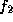to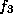, See Figure 5, Block 2T) are given by: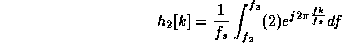(Eq. 3)

The factor of 2 is inserted to preserve the signal power. Once evaluated this becomes: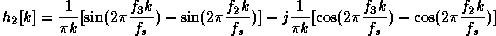(Eq. 4)

Notice that these filter tap coefficients have the form of an analytic signal (Eq. 2, with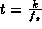), and that the real part of Eq. 4 is of the same form as the filter tap coefficients derived for Filter #1 (Eq 1).

In summary, the output of Filter #2 contains only positive frequency components (an analytic signal) which may be shifted in frequency. After frequency shifting, the negative frequencies may be restored by keeping only the real part of this analytic signal.

Note: The only differences between Filter #2T and Filter #2R are the frequencies used to develop the filter coefficients. Filter #2T is used in transmit mode and its pass-band output is shifted down in the spectrum. Filter #2R is used in receive mode and its pass-band output is shifted up in the spectrum.

Frequency Shifting

Frequency shifting can be done quite simply by multiplying a signal in the time domain by a complex exponential. As evidence of this, if the Fourier transform is taken of this product it can be seen that there will be a shift of frequencies in the frequency domain. This is shown below: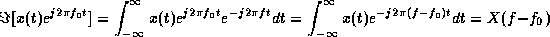(Eq. 5)

Note that t is time andis the number of Hertz shifted.

At first glance, it would appear that simply filtering the pass-band as in Filter #1 and then multiplying by this exponential would shift the frequencies to the proper place. But this will not work because the filtered signal contains both positive and negative frequency components. If a shift is attempted in this case, all frequency components will be shifted in a given direction and the resulting positive and negative frequency components will not be symmetrical around the origin. The frequency spectrum must be symmetrical around the origin, otherwise you have a complex number in the time domain (which you'll find rather difficult to send out the D/A converter).

The way around this problem is to create an analytic signal (as described in the previous section). This analytic signal can be frequency shifted to the desired location by multiplying by the complex exponential. Then, since it is still an analytic signal, the negative frequency components can be restored by taking its real part. This is how we shift the frequency spectrum while keeping it symmetrical around the origin.

The two different implementations of Filter #2 will be frequency shifted in different directions. In the transmitting section the upper band of frequencies (Figure 5, Block 2T) will be shifted down, adjacent to the lower band. As pointed out earlier this can be easily accomplished by multiplying the analytic signal output from Filter #2T by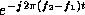, whereis how many Hertz you want it shifted down. Taking only the real part of this product effectively restores all of the negative frequency components of the shifted band, thus completing the shift operation. Adding this result to the output from Filter #1 yields the final output which can then be sent to the D/A converter and on to the transmitter.

In the receiver section the upper band of frequencies (Figure 5, Block 2R) will be shifted back up to their original place (Figure 5, Block 2T). This is accomplished by multiplying the output of Filter #2R by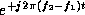. Again the real part of this product is taken and added to the output of Filter #1. This result is then sent to the D/A converter and on to the speaker. Notice that the only difference between transmitting and receiving is a sign change in the exponential shift operator.

## Implementation

Implementing the baseband compander system on a DSP brings many additional considerations into play above and beyond the theoretical aspects. In our implementation we used Texas Instruments DSP Starter Kit (the DSK). The DSK is limited to integer math, 1568 words of memory which may be split between data and program memory in a variety of ways , and a 100 nanosecond cycle time. Our sampling rate was 10 kHz and we were able to implement 100 tap FIR filters in real time.

When coding, it was important to minimize the number of instructions in order to leave as much memory as possible for the filter tap-coefficient tables and the complex exponential table. Wherever possible, we attempted to minimize the complex math and the number of arrays that had to be stored. We also conserved code by using much of the same code for transmitting as well as receiving. Filter #1 is the same for transmitting and receiving and Filter #2 is the same also except that different filter coefficients are used for transmitting and receiving. These facts can be used to shorten the code.

The filter coefficients were generated with the help of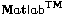Version 4.0. After the numbers were obtained and windowed (with Kaiser windowing), they were converted into integers by multiplying by large scale factors so that integer math could be used. They were also scaled so that the amplitude of both filters in the pass-band was equal. Finally, the numbers were written to files and included in the program memory and data memory.

We utilized the periodicity of the sine and cosine functions to develop a finite length look-up table for the complex exponential used in frequency shifting. Strictly speaking, this limits the sampling frequency,to be an integer multiple of the shift frequency,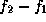. By sampling at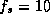kHz, and shifting the upper frequency band by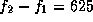Hz, we came up with an exponential lookup table containing 32 words. Actually, two separate lookup tables of 16 words were used, one for the sine function (imaginary part) and one for the cosine function (real part). For higher sampling rates the size of the tables will increase accordingly.

Figure 6 shows the pseudo code for the DSP program we developed. Actual assembly code and filter coefficients that we used for the DSK can be obtained over the Internet by anonymous ftp here

## Conclusions

The prototype system we developed works surprisingly well with quite understandable speech even after all the processing that has been done to it. We tested transmissions with two companders (DSKs) on 40 meter SSB using three ham radios to compare co-channel interference and signal quality. The third radio was used for creating an interference signal. The compander definitely helped to lessen the co-channel interference from this interfering signal transmitting at the same signal strength. We were unable to test the full impact of the compander, though, since we have only 2 DSKs. In other words the interfering radio couldn't transmit a companded signal. We have not used it extensively yet on the air since our present prototype does not have automatic transmit/receive mode switching, or stand-alone capability.

## Cited References

 Richard W. Harris & J.F. Cleveland, "A Baseband Communications System", QST, November 1978.

 Alan V. Oppenheim & Ronald W. Schafer, Discrete-Time Signal Processing, Prentice Hall, 1989.

 John H. Karl, An Introduction to Digital Signal Processing, Academic Press, Inc., 1989.

 Ronald N. Bracewell, The Fourier Transform and Its Applications, 2nd Ed., 1986.

 TMS 320C2X User's Guide - Rev. C, Texas Instruments Inc., 1993.

 Frank P. Morrison, "The Magic of Digital Filtering", QEX, February 1993.